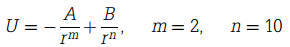### Create an Account

Home / Questions / The potential energy U of two atoms a distance r apart is Given that the atoms form a s...

# The potential energy U of two atoms a distance r apart is Given that the atoms form a stable molecule at a separation of 0.3 nm with an energy of 4 eV calculate A and B. Also find the force

The potential energy U of two atoms, a distance r apart, isGiven that the atoms form a stable molecule at a separation of 0.3 nm with an energy of 4 eV, calculate A and B. Also find the force required to break the molecule, and the critical separation at which the molecule breaks. You should sketch an energy/distance curve for the atom, and sketch beneath this curve the appropriate force/distance curve.

May 16 2020 View more View LessGet Solution